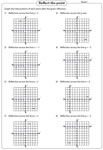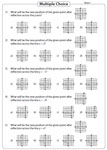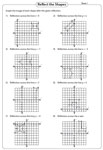Reflection Worksheets

Reflection worksheets have exclusive pages to understand the concepts of reflection and symmetry. Exercises to graph the images of figures across the line of reflection, reflection of points and shapes are here for practice. In addition, skills to write the coordinates of the reflected images and more are in these worksheets.

Printing Help - Please do not print reflection worksheets directly from the browser. Kindly download them and print.

Reflection across the axes

In these reflection worksheets the figure and a line of reflection are given in each problem. Draw the image obtained after reflection.Draw the other half: Mirror image

Draw the other half of the symmetrical shape. Practice these pages to know the relationship between reflection and symmetry.Reflection of a point

In these worksheets reflect the given point and graph the image across the axes and across x=a, y=b, where a and b are parameters.Choose the correct reflection

Choose the correct option which is the reflection of the given point. Reflect the point across the line of reflection.Reflection of shapes

In these reflection worksheets reflect the shapes across the lines of reflection. Each worksheet has eight problems.Reflection of triangles

Reflect each triangle and draw its image on the grid following the given rule (across the axes; x=a; y=b) shown above each grid.Reflect each quadrilateral across the given line of reflection. Graph the image on the grid and label them.Write the rules

Write a rule to describe each reflection. Example: Reflection across the x-axis or the y-axis or across a line.Writing coordinates: With graph

Graph the image of each figure after the given reflection. Label the image and write the coordinates.Writing coordinates

Observe the given coordinates carefully. Write the new coordinates obtained after reflection in these worksheets.The entire collection of worksheets on reflection can be downloaded in a jiffy!

Related Worksheets